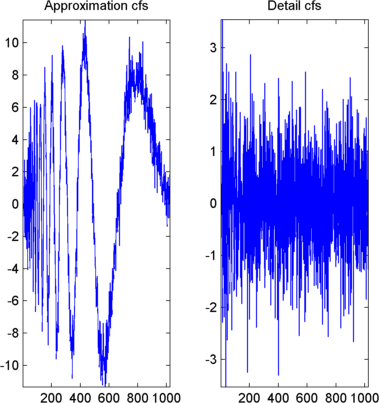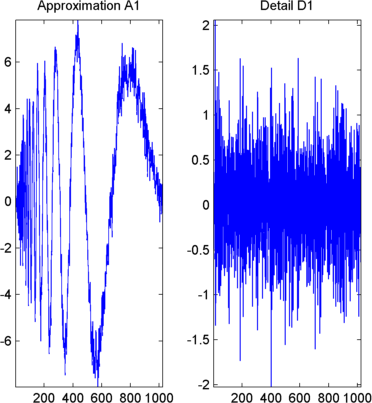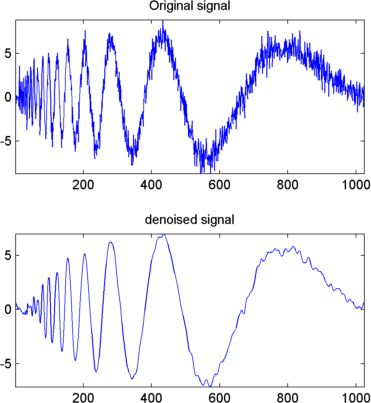## 1-D Stationary Wavelet Transform

This topic takes you through the features of 1-D discrete stationary wavelet analysis using the Wavelet Toolbox™ software. For more information see Nondecimated Discrete Stationary Wavelet Transforms (SWTs) in the Wavelet Toolbox User's Guide.

The toolbox provides these functions for 1-D discrete stationary wavelet analysis. For more information on the functions, see the reference pages.

Function Name

Purpose

`swt`

Decomposition

### Synthesis-Reconstruction Functions

Function Name

Purpose

`iswt`

Reconstruction

The stationary wavelet decomposition structure is more tractable than the wavelet one. So the utilities, useful for the wavelet case, are not necessary for the stationary wavelet transform (SWT).

In this section, you'll learn to

• Perform a stationary wavelet decomposition of a signal

• Construct approximations and details from the coefficients

• Display the approximation and detail at level 1

• Regenerate a signal by using inverse stationary wavelet transform

• Perform a multilevel stationary wavelet decomposition of a signal

• Reconstruct the level 3 approximation

• Reconstruct the level 1, 2, and 3 details

• Reconstruct the level 1 and 2 approximations

• Display the results of a decomposition

• Reconstruct the original signal from the level 3 decomposition

• Remove noise from a signal

Since you can perform analyses either from the command line or using the Wavelet Analyzer app, this section has subsections covering each method.

The final subsection discusses how to exchange signal and coefficient information between the disk and the graphical tools.

### 1-D Analysis Using the Command Line

This example involves a noisy Doppler test signal.

From the MATLAB® prompt, type

```load noisdopp ```
2. Set the variables. Type

```s = noisdopp; ```

For the SWT, if a decomposition at level `k` is needed, `2^k` must divide evenly into the length of the signal. If your original signal does not have the correct length, you can use the `wextend` function to extend it.

3. Perform a single-level Stationary Wavelet Decomposition.

Perform a single-level decomposition of the signal using the `db1` wavelet. Type

```[swa,swd] = swt(s,1,'db1'); ```

This generates the coefficients of the level 1 approximation (swa) and detail (swd). Both are of the same length as the signal. Type

```whos ```
NameSizeBytesClass
`noisdopp``1x1024``8192``double array`
`s``1x1024``8192``double array`
`swa``1x1024``8192``double array`
`swd``1x1024``8192``double array`
4. Display the coefficients of approximation and detail.

To display the coefficients of approximation and detail at level 1, type

```subplot(1,2,1), plot(swa); title('Approximation cfs') subplot(1,2,2), plot(swd); title('Detail cfs') ```5. Regenerate the signal by Inverse Stationary Wavelet Transform.

To find the inverse transform, type

```A0 = iswt(swa,swd,'db1'); ```

To check the perfect reconstruction, type

```err = norm(s-A0) err = 2.1450e-14 ```
6. Construct and display approximation and detail from the coefficients.

To construct the level 1 approximation and detail (`A1` and `D1`) from the coefficients `swa` and `swd`, type

```nulcfs = zeros(size(swa)); A1 = iswt(swa,nulcfs,'db1'); D1 = iswt(nulcfs,swd,'db1'); ```

To display the approximation and detail at level 1, type

```subplot(1,2,1), plot(A1); title('Approximation A1'); subplot(1,2,2), plot(D1); title('Detail D1'); ```7. Perform a multilevel Stationary Wavelet Decomposition.

To perform a decomposition at level 3 of the signal (again using the `db1` wavelet), type

```[swa,swd] = swt(s,3,'db1'); ```

This generates the coefficients of the approximations at levels 1, 2, and 3 (`swa`) and the coefficients of the details (`swd`). Observe that the rows of `swa` and `swd` are the same length as the signal length. Type

```clear A0 A1 D1 err nulcfs whos ```
NameSizeBytesClass
`noisdopp``1x1024``8192``double array`
`s``1x1024``8192``double array`
`swa``3x1024``24576``double array`
`swd``3x1024``24576``double array`
8. Display the coefficients of approximations and details.

To display the coefficients of approximations and details, type

```kp = 0; for i = 1:3 subplot(3,2,kp+1), plot(swa(i,:)); title(['Approx. cfs level ',num2str(i)]) subplot(3,2,kp+2), plot(swd(i,:)); title(['Detail cfs level ',num2str(i)]) kp = kp + 2; end ```9. Reconstruct approximation at Level 3 From coefficients.

To reconstruct the approximation at level 3, type

```mzero = zeros(size(swd)); A = mzero; A(3,:) = iswt(swa,mzero,'db1'); ```
10. Reconstruct details from coefficients.

To reconstruct the details at levels 1, 2 and 3, type

```D = mzero; for i = 1:3 swcfs = mzero; swcfs(i,:) = swd(i,:); D(i,:) = iswt(mzero,swcfs,'db1'); end ```
11. Reconstruct and display approximations at Levels 1 and 2 from approximation at Level 3 and details at Levels 2 and 3.

To reconstruct the approximations at levels 2 and 3, type

```A(2,:) = A(3,:) + D(3,:); A(1,:) = A(2,:) + D(2,:); ```

To display the approximations and details at levels 1, 2 and 3, type

```kp = 0; for i = 1:3 subplot(3,2,kp+1), plot(A(i,:)); title(['Approx. level ',num2str(i)]) subplot(3,2,kp+2), plot(D(i,:)); title(['Detail level ',num2str(i)]) kp = kp + 2; end ```12. Remove noise by thresholding.

To denoise the signal, use the `ddencmp` command to calculate a default global threshold. Use the `wthresh` command to perform the actual thresholding of the detail coefficients, and then use the `iswt` command to obtain the denoised signal.

### Note

All methods for choosing thresholds in the 1-D Discrete Wavelet Transform case are also valid for the 1-D Stationary Wavelet Transform, which are also those used by the Wavelet Analysis app. This is also true for the 2-D transforms.

```[thr,sorh] = ddencmp('den','wv',s); dswd = wthresh(swd,sorh,thr); clean = iswt(swa,dswd,'db1'); ```

To display both the original and denoised signals, type

```subplot(2,1,1), plot(s); title('Original signal') subplot(2,1,2), plot(clean); title('denoised signal') ```The obtained signal remains a little bit noisy. The result can be improved by considering the decomposition of `s` at level 5 instead of level 3, and repeating steps 14 and 15. To improve the previous denoising, type

```[swa,swd] = swt(s,5,'db1'); [thr,sorh] = ddencmp('den','wv',s); dswd = wthresh(swd,sorh,thr); clean = iswt(swa,dswd,'db1'); subplot(2,1,1), plot(s); title('Original signal') subplot(2,1,2), plot(clean); title('denoised signal') ```A second syntax can be used for the `swt` and `iswt` functions, giving the same results:

```lev = 5; swc = swt(s,lev,'db1'); swcden = swc; swcden(1:end-1,:) = wthresh(swcden(1:end-1,:),sorh,thr); clean = iswt(swcden,'db1'); ```

You can obtain the same plot by using the same plot commands as in step 16 above.

### Interactive 1-D Stationary Wavelet Transform Denoising

Now we explore a strategy to denoise signals, based on the 1-D stationary wavelet analysis using the Wavelet Analyzer app. The basic idea is to average many slightly different discrete wavelet analyses.

1. Start the Stationary Wavelet Transform Denoising 1-D Tool.

From the MATLAB prompt, type `waveletAnalyzer`.

The Wavelet Analyzer appears.Click the SWT Denoising 1-D menu item. The discrete stationary wavelet transform denoising tool for 1-D signals appears.

At the MATLAB command prompt, type

`load noisbloc;`
In the SWT Denoising 1-D tool, select File > Import Signal from Workspace. When the Import from Workspace dialog box appears, select the `noisbloc` variable. Click OK to import the noisy blocks signal.

3. Perform a Stationary Wavelet Decomposition.

Select the `db1` wavelet from the Wavelet menu and select 5 from the Level menu, and then click the Decompose Signal button. After a pause for computation, the tool displays the stationary wavelet approximation and detail coefficients of the decomposition. These are also called nondecimated coefficients since they are obtained using the same scheme as for the DWT, but omitting the decimation step (see Fast Wavelet Transform (FWT) Algorithm in the Wavelet Toolbox User's Guide).4. denoise the signal using the Stationary Wavelet Transform.

While a number of options are available for fine-tuning the denoising algorithm, we'll accept the defaults of fixed form soft thresholding and unscaled white noise. The sliders located on the right part of the window control the level-dependent thresholds, indicated by yellow dotted lines running horizontally through the graphs of the detail coefficients to the left of the window. The yellow dotted lines can also be dragged directly using the left mouse button over the graphs.

Note that the approximation coefficients are not thresholded.

Click the denoise button.The result is quite satisfactory, but seems to be oversmoothed around the discontinuities of the signal. This can be seen by looking at the residuals, and zooming on a breakdown point, for example around position 800.#### Selecting a Thresholding Method

Select hard for the thresholding mode instead of soft, and then click the denoise button.

The result is of good quality and the residuals look like a white noise sample. To investigate this last point, you can get more information on residuals by clicking the Residuals button.

### Importing and Exporting from the Wavelet Analysis App

The tool lets you save the denoised signal to disk. The toolbox creates a MAT-file in the current folder with a name of your choice.

To save the above denoised signal, use the menu option File > Save denoised Signal. A dialog box appears that lets you specify a folder and filename for storing the signal. Type the name `dnoibloc`. After saving the signal data to the file `dnoibloc.mat`, load the variables into your workspace:

```load dnoibloc whos ```
NameSizeBytesClass
`dnoibloc``1x1024``8192``double array`
`thrParams``1x5``580``cell array`
`wname``1x3``6``char array`

The denoised signal is given by `dnoibloc`. In addition, the parameters of the denoising process are available. The wavelet name is contained in `wname`:

```wname wname = db1 ```

and the level dependent thresholds are encoded in `thrParams`, which is a cell array of length 5 (the level of the decomposition). For i from 1 to 5, `thrParams{i}` contains the lower and upper bounds of the interval of thresholding and the threshold value (since interval dependent thresholds are allowed). For more information, see 1-D Adaptive Thresholding of Wavelet Coefficients.

For example, for level 1,

```thrParams{1} ans = 1.0e+03 * 0.0010 1.0240 0.0041 ```

Here the lower bound is 1, the upper bound is 1024, and the threshold value is 4.1. The total time-interval is not segmented and the procedure does not use the interval dependent thresholds.

##### SupportGet trial now